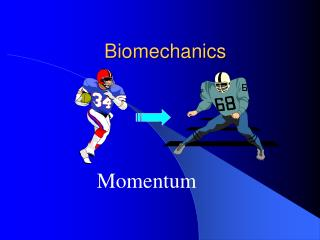DownloadDownload PresentationBiomechanics

# Biomechanics

Download Presentation## Biomechanics

- - - - - - - - - - - - - - - - - - - - - - - - - - - E N D - - - - - - - - - - - - - - - - - - - - - - - - - - -
##### Presentation Transcript

1. Biomechanics Momentum

2. Today’s session will cover • What is momentum • Types of momentum • - Linear momentum • - Angular momentum

3. Momentum. • Momentum is moving inertia. It is a product not only of an objects mass but also how fast it is moving. • We can rewrite Newton’s 1st law to include momentum: an object that is moving will continue to move in the direction the force was applied, until another force is applied.

4. Types of momentum. • There are two types of momentum: • -Linear • -Angular • Linear Momentum: When an object translates, it has momentum. • The equation for linear momentum is as follows: • Momentum = mass x velocity • Mo(kg/ms-1) = m(kgs) x v(ms-1).

5. Angular momentum example • Figure out which of the following objects has the greatest momentum, using the formula for linear momentum: • Object A = Jonah Lomu with a mass of 120kgs, and is moving at 10m/s • Object B = George Gregan with a mass of 78kgs, moving at 15m/s

6. Jonah Mo = m x v. Mo = 120 x 10 Mo = 1200 kgm/s George Gregan Mo = m x v. Mo = 78 x 15 Mo = 1170kgm/s Angular momentum answer ‘Therefore Jonah has more momentum’

7. Angular momentum • When an object rotates, it has momentum. • In angular momentum, inertia has an important role to play. • In rotation movements, inertia is due not only to mass but bow mass is distributed about the axis of rotation.

8. Angular momentum • Consider an ice skater spinning. How do they speed themselves up in the spin? How do they slow themselves down? • To speed up, they bring their body parts closer to the axis of rotation then, move them out again to slow down. • In pairs try and come up with three examples.

9. These situations provide us with the two key principles regarding angular momentum. • Delete the incorrect option in each case. • When the mass is moved closer to the axis of rotation, inertia increases / decreases, and angular velocity increases / decreases. As a consequence the body spins faster. • When the mass is moved further away from the axis of rotation, inertia increases / decreases and angular velocity increases / decreases. As a consequence the body spins slower.

10. Other sporting examples of this principle in action include: • 1. Pirouettes in ballet • 2. Somersaults in tumbling • 3. Springboard diving moves We can now apply these principles to actual sporting situations in order to explain what is happening, or maybe why certain events have happened. Plot the change in inertia and angular velocity as the gymnast below performs a headstand to forward roll.

11. Explain in biomechanical terms the relationship that occurs between moment of inertia and angular velocity as the gymnast performs the headstand to forward roll. As the gymnast tucks from A to C, the mass is brought closer to the axis of rotation. As a consequence inertia decreases and angular velocity (speed of roll) increases. As gymnast opens out from C to E, the mass is moved further from the axis of rotation. Inertia increases, angular velocity decreases.

12. Let us assume that the gymnast is unable to stand at the end of performing the skill, instead they fall backwards. Explain in biomechanical detail what may have happened for this to occur. They opened out too early (inertia increases, angular velocity decrease). I.e. they don’t have enough speed of rotation to get to the standing position.

13. Conservation of Momentum • In any system, momentum is covered. The Law of Conservation of Momentum states: When objects collide, momentum is conserved throughout. • We will use a baseball game with a pitcher throwing the ball at a batter as an example. The total momentum before impact (before the ball is hit) is: equal to the momentum of the bat and the momentum of the ball.

14. After impact, the total momentum of the bat and ball is still the same, except some of the momentum of the bat has been transferred to the ball. This is because momentum is always conserved. • Basically – Momentum before impact = Momentum after impact

15. Using this concept, explain why: • A. The white ball in pool slows down after impacting the black. Some of the momentum of the white ball is passed onto the black ball • B. When suddenly braking in a car, your body moves forward (thankfully you are wearing a seatbelt). The momentum of the car is transferred to your body. This is why object in a car are propelled forward on braking.